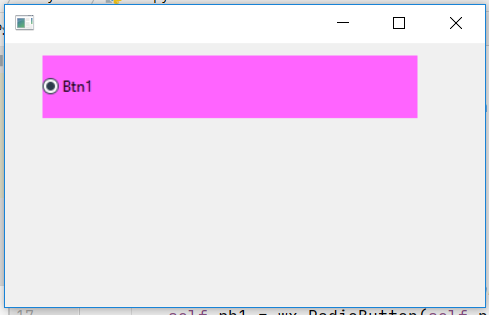# wxPython – Get size of Radio Button Window

In this article we are going to lear that how can we get the size of Radio button window present in the frame. In order to do this we will use GetSize() function associated with wx.RadioButton class of wxPython. GetSize() function simply returns the size of the radio button in pixels.
GetSize() function require no arguments.

Parameters: GetSize() function require no arguments.

Return Type: wx.Size

Returns: Returns the size of the radio button

Code Example:

 `import` `wx ` ` `  `APP_EXIT ``=` `1` ` `  ` `  `class` `Example(wx.Frame): ` ` `  `    ``def` `__init__(``self``, ``*``args, ``*``*``kwargs): ` `        ``super``(Example, ``self``).__init__(``*``args, ``*``*``kwargs) ` ` `  `        ``self``.InitUI() ` ` `  `    ``def` `InitUI(``self``): ` `        ``self``.pnl ``=` `wx.Panel(``self``) ` `         `  `        ``# create radio button at position (30, 10) ` `        ``self``.rb1 ``=` `wx.RadioButton(``self``.pnl, label ``=``'Btn1'``, ` `                             ``pos ``=``(``30``, ``10``), size ``=``(``100``, ``20``)) ` ` `  `        ``# change background colour ` `        ``self``.rb1.SetBackgroundColour((``255``, ``100``, ``255``, ``255``)) ` ` `  `        ``# change size to (300, 50) ` `        ``self``.rb1.SetSize((``300``, ``50``)) ` ` `  `        ``# print size of radio button ` `        ``print``(``self``.rb1.GetSize()) ` ` `  ` `  `def` `main(): ` `    ``app ``=` `wx.App() ` `    ``ex ``=` `Example(``None``) ` `    ``ex.Show() ` `    ``app.MainLoop() ` ` `  ` `  `if` `__name__ ``=``=` `'__main__'``: ` `    ``main() `

Console Output:

```(300, 50)
```

Output Window:My Personal Notes arrow_drop_upCheck out this Author's contributed articles.

If you like GeeksforGeeks and would like to contribute, you can also write an article using contribute.geeksforgeeks.org or mail your article to contribute@geeksforgeeks.org. See your article appearing on the GeeksforGeeks main page and help other Geeks.

Please Improve this article if you find anything incorrect by clicking on the "Improve Article" button below.

Article Tags :

Be the First to upvote.

Please write to us at contribute@geeksforgeeks.org to report any issue with the above content.